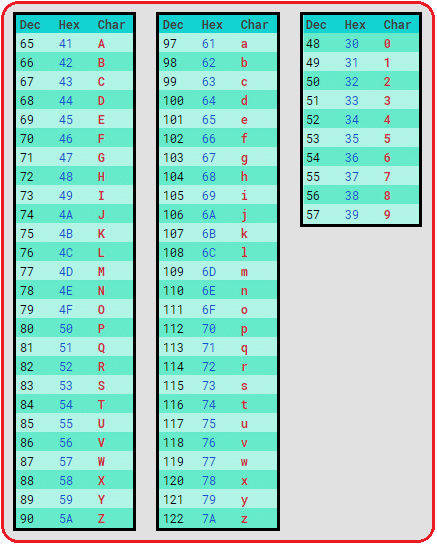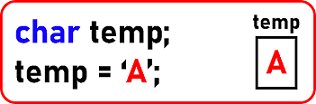Introduction to Strings in C

Introduction to Strings in C Language:

In this article, we will learn about Strings in C Language. We have discussed about arrays in our previous section. In this article, we will discuss introduction of strings in the C Language. We will cover the following topics:

1. Character set / ASCII codes
2. Character Array
3. String
4. Creating a String

Character SET / ASCII Codes in C Language:

Character set is the set of characters that are supported by a programming language like C, C++ or any other language. So, the set of characters supported by a programming language will be same as the set of characters that are supported by any computer system. We know that the computer system works on a binary number system. So, everything in computer is numbers then how they can support characters? So basically, they don’t support characters.

Then how we make them work on characters? We will define some set of numbers as characters. So, it means for every character we define some numeric value. So, for the English alphabet, for every character there are some codes defined and those codes are standard codes. Every electronic machine follows that same set of codes and those codes are called as American Standard Code for Information Interchange. That are ASCII codes. These codes are given by American National Standards Institute that is ANSI and also it is ISO standard.

So, there is a reason that every electronic device supposedly is called for English language and moreover, for other national languages like a Chinese, Japanese or Hindi, the codes are defined and those are ISO standard codes and those schools are called us UNICODES. So, we will first discuss about ASCII codes then we will discuss a little bit about Unicode also.

ASCII Codes in C Language:

ASCII codes are for English language. Now how the codes are defined? For every letter of character there is a code available. Below is the list of some ASCII Codes:So, these are uppercase letters codes are starting from 65 (A) up to 90 (Z), lowercase letters codes are from 97 (a) up to 122 (z) and numbers are starting from 0 (48) up to 9 (57).

Now basically all the symbols that you find on keyboard that forms a character set and for every symbol on the keyboard there is some ASCII code available now the other symbols are remaining like special characters i.e. *, %, \$, (, ), [, ], !, ~… There are ASCII codes for special characters.

Generally, we work with the alphabets, numbers and some special characters which we have written above.

I have written the ASCII codes and you should remember these ASCII codes for uppercase, lowercase as well as these numeric symbols and also for ENTER ASCII code is 10, SPACEBAR ASCII code is 13 and ESCAPE ASCII code is 27. So, these may also be helpful if you can remember them.

From where ASCII codes are starting and where they are ending is important. Total 128 ASCII codes are there. Starting from 0 to 127. To represent these ASCII code, any one symbol, 7 bits are sufficient that is binary bits.

Unicode in C Language:

Now let’s discuss about Unicode. Unicode are for all the languages so ASCII Code become the subset of Unicode. Like English is also one of the languages so it becomes subset of Unicode. Unicode takes 2 bytes of memory that is 16 bits. Because it is supporting all national languages and these 16 bits can be represented in the form of hexadecimal codes. Hexadecimal codes are represented in 4 bits so Unicode are represented in 4×4 bits hexadecimal that is 16 bits.

So, these Unicode are represented in the 4 digits of hexadecimal, like for example C03A. So, Unicode are represented in the form of hexadecimal. You can go to a website – Unicode.org. There you can find the code for various languages.

Character Array in C Language:

Now let us understand how a character is represented and what is character array. Let us see how to declare a character type variable in C and C+ +:char is a data type and we declare a variable name as a temp. It takes one bite of the memory. So, temp takes just one bite. And if we want to store something then we can initialize it with the character i.e. A. For giving a character constant, it must be in the single quotes and we can give only single alphabet:So, we should have only one single alphabet inside single quotes then it will be acceptable. Now what actually is stored in the memory? Actually, inside the memory value 65 is stored. It’s not ‘A’. ‘A’ is not represented in computer memory. To print this ‘A’, we simply write:Here printf will print 65 but we have given our control character as ‘%c’ so it will print A on the screen and if I make it as a ‘%d’ then decimal number that is integer type and it will display 65 on the screen.

Character Array:

We will create our array of characters. For creating an array of characters just like any other. We will take array name as ‘B’ of size 5.Now getting initialized this one.This is a declaration plus initialization. So an array will be created with the name B. And it will have alphabets.This is how we can create an array without giving any size. So same type of array will be created of size 5 and initialized with all these alphabets we haven’t mentioned the size. It will be taken depending on the number of alphabets we’re assigning.And one more method we can create an array by either mentioning or not mentioning the size. So, these are the ASCII code for these alphabets.We will create one more array and we will mention only two alphabets. So now the array is created with only ‘a’ and ‘b’ alphabet are restored.

So, the set of characters are still here but the array size is total five. But we have only two valid alphabets rest of the places are empty / vacant not in use. Now next we will take the same example and we will explain you what are strings.

Strings in C Language:

We want to store a name in an array so we will create an array of characters of name ‘boy’ and give size as 10 and here we will store ‘Rohan’:It’s a string for storing names for storing the words or sentences of paragraphs. String is nothing but a set of characters. So a name of boy or anything is a string. Now the problem is:See here the size of an array is 10 but the string size is only 4 alphabets. Then how do I know where this string is ending? So that is the important thing. When the size of an array may be larger but you are having only part of it as a string then we need to know till where we have a string.

So, we should know that the length of a string or we should have the end point of a string. So yes, in C and C++ it is marked it null character that is ‘\0’. ‘\0’ this is a null symbol. We can also say that a string delimiter or end of the string or null character or string terminator. This is use to show the end of string.

So, in C or C++ strings are terminated with null character that is ‘\0’. But whereas in other language like Java strings will not have ‘\0’.

Then how to know how many alphabets are valid.\? So that is known with the help of length. In Java, String length is known or the size of the string is known by its length but in C or C++ the size of a string is known by finding a termination character that is null character so strings are delimited by ‘\0’.Now this is just an array of characters. How to make it as a string in C / C++? We must write ‘\0’ also.Now this becomes a string. Without ‘\0’ it is just an array of characters. This is the difference between array of characters and a string.

Now let’s see what are the methods for creating or declaring a string and also initializing it. Above is the 1st method for declaring as well as initializing a string. 2nd method we can declare a string without any size and we will use same name:Then what will be the size of this array? The size of this array is 6 so for storing five alphabets of a name and also provide a space for ‘\0’ as it also consumes memory. Then next method of declaring or initializing a string is:We can write down the name in double quotes. So only ‘ROHAN’ is written in double quotes so ‘\0’ will be automatically included. So then this looks better than these two methods. One more method of creating a string is:This is a character pointer. Then where does the string will be created? This string will be automatically created in heap. Though we did not use a malloc () function or we did not write a new but this is implicitly allocated in heap memory. The array created by above methods will be created inside the stack.Inside heap ‘y’ is pointing to the array which is directly accessible to a program. This is created in heap so this indirectly accessible using a pointer. Automatically compiler will create this string inside heap and pointer will point there. Now let we discuss about printing a string.For printing the above string:So ‘%s’ is a control character for string. We can just give the name of an array and string will be displayed. Remember it is not possible for any other type of array, like for integer or float. If suppose we want to read some new name another name and then here, we will use ‘scan’:scanf can also read strings from the keyboard and store those alphabets there followed by ‘\0’. 0 or so but indefinite scan if both are dependent on that slab zero Faldo library functions of C language that are meant for strings are dependent on slash 0.

In the next article, I am going to discuss String in C Language with Examples. Here, in this article, I try to give a brief Introduction to Strings in C Language. I hope you enjoy this Introduction to Strings in C Language article. I would like to have your feedback. Please post your feedback, question, or comments about this Introduction to Strings in C Language article